Lei, J., Ren, D., Liu, Z., Wang, S., Lei, D., Luo, J., and Lei, M. (2023). “Discrete element contact parameters measurement and calibration of sugarcane leaves based on RSM-PSO,” BioResources 18(3), 4994-5012.

#### Abstract

In response to the lack of accurate and reliable parameters in the discrete element simulation analysis of the sugarcane leaf crushing and return device, in this work, the actual and simulated errors of two stacking angles α and β of sugarcane leaves were used as indicators to calibrate the discrete element parameters. The second-order regression models between the important parameters and the indicators were obtained by Plackett-Burman test, steepest climb test, and Box-Behnken optimization test, and the analysis of variance and interaction factors were performed. The response surface method and particle swarm optimization algorithm were used to find the best significance parameters, and the best combination of significance parameters was obtained: the static friction coefficient between sugarcane leaves was 0.306, the rolling friction coefficient between sugarcane leaves was 0.198, and the recovery coefficient of sugarcane leaf-plate collision was 0.102. The relative errors of the simulation results and the physical test stacking angle α and stacking angle β were 0.609% and 1.643%, respectively. The calibration parameters can provide a theoretical reference for the design and research of sugarcane leaf crushing and returning machines, as well as the calibration of discrete element model parameters for leaf crops with high water content.

Discrete Element Contact Parameters Measurement and Calibration of Sugarcane Leaves Based on RSM-PSO

Junle Lei,* Danni Ren, Zhaochong Liu, Shuaiwei Wang, Dingyuan Lei, Jiawei Luo, and Ming Lei

In response to the lack of accurate and reliable parameters in the discrete element simulation analysis of the sugarcane leaf crushing and return device, in this work, the actual and simulated errors of two stacking angles α and β of sugarcane leaves were used as indicators to calibrate the discrete element parameters. The second-order regression models between the important parameters and the indicators were obtained by Plackett-Burman test, steepest climb test, and Box-Behnken optimization test, and the analysis of variance and interaction factors were performed. The response surface method and particle swarm optimization algorithm were used to find the best significance parameters, and the best combination of significance parameters was obtained: the static friction coefficient between sugarcane leaves was 0.306, the rolling friction coefficient between sugarcane leaves was 0.198, and the recovery coefficient of sugarcane leaf-plate collision was 0.102. The relative errors of the simulation results and the physical test stacking angle α and stacking angle β were 0.609% and 1.643%, respectively. The calibration parameters can provide a theoretical reference for the design and research of sugarcane leaf crushing and returning machines, as well as the calibration of discrete element model parameters for leaf crops with high water content.

DOI: 10.15376/biores.18.3.4994-5012

Keywords: Sugarcane leaves; Discrete elements; Stacking angle; Optimized design

Contact information: College of Mechanical and Control Engineering, Guilin University of Technology, Guilin, 541004, P. R. China; *Corresponding author: 40911409@qq.com

INTRODUCTION

Sugarcane occupies a dominant position among sugar crops and cash crops in China, and it is widely concentrated in Guangxi, Guangdong, and Hainan, where it accounts for more than 85% of the year-round sugar crop area in China (Fan et al. 2020). Sugarcane leaves are often left in large quantities after harvesting at maturity, and most of them are burned on site, which not only wastes resources but also pollutes the environment. Crushing and returning the leaves to the field can significantly improve the physical properties of the soil, enhance the soil’s ability to retain water and fertilizer, and supply nutrients, thus promoting the growth and increasing the yield of sugarcane (Wei et al. 2011). Thus, its resource utilization is imperative.

As sugarcane leaves are characterized by large volume, toughness, and high water content (Wei et al. 2022), their material properties are quite different from those of other crops, and the crushing process is prone to uneven crushing and fiber entanglement, which seriously restricts the application and promotion of mechanized sugarcane leaf crushing and field return technology. With the rapid development of computer technology, EDEM, a numerical simulation software based on the discrete element method, has been widely used in the field of agricultural equipment research (Ghodki et al. 2019). In the process of sugarcane leaf crushing work, the interaction relationship between sugarcane leaf particles is complex. The objective and accurate measurement of discrete element simulation parameters can help to deeply study its crushing mechanism, making it possible to optimize the parameters and performance of the crushing mechanism in the sugarcane leaf crushing and returning machine. The discrete element simulation parameters mainly include intrinsic parameters (Poisson’s ratio, density, shear modulus, etc.) and contact parameters (impact recovery coefficient, static friction coefficient, rolling friction coefficient, etc.). Most of the intrinsic parameters of the discrete element model are consistent with the values of the real parameters, but due to the errors of the particle model and the complex contact characteristics between the particles, some of the contact parameters should be recalibrated and optimized (Horabik and Molenda 2016).

The discrete element contact parameters of different granular materials have been carried out and calibrated by domestic and foreign scholars. Tong et al. (2023) established an EDEM simulation model of corn stover built with and without stalk nodes and investigated the mechanical properties of the outer skin and inner flesh of corn stover for physical and simulation tests. Fan et al. (2022) constructed a simulation model of a typical pair using a combined Hertz-Mindlin model. The simulation parameters between pears and contact materials (PVC, EVA foam material) were used to calibrate the simulation parameters of the intercourse material (PVC, EVA foam material) and the optimal parameter combination was used to obtain the optimal parameter combination. Qiu et al. (2022) used cinnamon soil as the research object, determined the material property parameters such as particle size distribution, Poisson’s ratio and shear modulus of cinnamon soil, and designed Plackett-Burman test, steepest climb test and Box-Behnken with stacking angle as the response value to calibrate and optimize the physical parameters of soil simulation. Zhang et al. (2022) calibrated maize single roots (SMR) and maize root-soil mixtures (MRSM) of different diameters by angle of repose (AOR) tests, and the relative errors of simulated and measured AOR and pixel peaks for the final stacking profiles of SMR and MRSM were less than 5%. The above relating studies can be used as a basis for the basic parameters of simulation.

In the process of developing sugarcane leaf crushing and returning implements, it is important to establish accurate and reliable discrete element models of sugarcane leaves. This will make it possible to analyze the interaction mechanism between sugarcane leaves and implements and to further develop the theory of sugarcane leaf crushing and returning technology. However, there are few studies related to the contact parameters of fresh leaves such as mature sugarcane leaves. Moreover, the existing studies on the calibration of discrete element model parameters seldom adopt the relative error values of two stacking angles as the test index nor do they use algorithms to solve the final results. In this work, the basic material characteristics parameters and contact parameters of crushed sugarcane leaves were measured by physical test methods, and the parameters with significant effects on the relative error values of the two stacking angles and their value ranges were screened by Plackett-Burman and steepest climb tests. The Box-Behnken test was used to calibrate the parameters with significant effects and to establish the regression equation. The response surface method and particle swarm optimization algorithm were used to find and calculate the significant parameters and obtain the optimal solution. This provides a reference for the design of sugarcane leaf crushing and returning machines and the parameter calibration of discrete element models for high moisture content leaf crops.

EXPERIMENTAL

Test Material

The test material was chosen from the Gui sugarcane No. 42 produced in November 2022 from Guilin Branch of Guangxi Academy of Agricultural Sciences (110°28′E, 25°28′N). In this work, 10 sugarcane leaves were randomly selected as samples. In order to facilitate the subsequent simulation of the crushing process in the sugarcane leaf return crushing mechanism using the discrete element method, the crushing process was performed using a sugarcane crusher after the impurities were removed from the sugarcane leaf samples. Some sugarcane leaves suffered different degrees of structural damage during the crushing process. To reduce the difficulty of EDEM model development and improve the computational efficiency, sugarcane leaf samples with relatively good shape were selected and collected, divided into five groups and then the sugarcane leaves were measured using an electronic vernier caliper (Deqing Shengtaixin Electronic Technology Co., Ltd., Huzhou, China) with an accuracy of 0.01 mm. The length, width, and thickness of the leaves were measured using an electronic vernier caliper with an accuracy of 0.01 mm. Shredded leaves with a length of about 15 mm to 30 mm were selected, and their density and moisture content were measured using the immersion and drying methods. The number of shredded leaves was found to be superior, occupying 86.7% of the total, and the number of finely shredded leaves was less, occupying 13.3% of the total. The properties of the samples are shown in Table 1.

Table 1. Physical Property Parameters of Shredded Sugarcane Leaves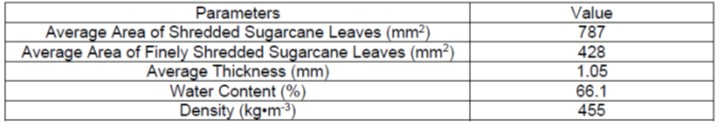Simulation Model

The simulation test was conducted with the average area of shredded sugarcane leaves (787 mm2) and the average area of finely shredded sugarcane leaves (428 mm2) and the average thickness value (1.05 mm), as shown in Table 1. The simulation models of shredded and finely shredded sugarcane leaves were randomly generated according to the standard values (mean value of 1 and standard deviation of 0.05), where the number of shredded and finely shredded sugarcane leaves ratio was 13:2, which was consistent with the measured data. Geometric models were first constructed based on the actual shapes of the shredded and finely shredded sugarcane leaf particles using SolidWorks (Dassault Systemes S.A, v.2020, Concord, MA, USA) based on the actual shape dimensions of the sugarcane leaf and finely sugarcane leaf particles. Then the 3D model of the particles was exported to IGS format and imported into EDEM software (DEM Solutions S.A, v.2020, Concord, MA, USA), and filled with the particle auto-fill tool to build the particle simulation model (Lei et al. 2022). The filled sugarcane leaf and finely sugarcane leaf particle models are shown in Fig. 1.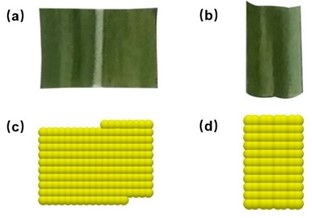Fig. 1. Actual particle model: (a) Shredded sugarcane leaf; (b) Finely shredded sugarcane leaf. Discrete element particle model: (c) Shredded sugarcane leaf; (d) Finely shredded sugarcane leaf

Crash Recovery Factor Measurement

The collision recovery coefficient is the ratio of the normal relative separation velocity between the contact points of two objects after the collision (v1) and before the collision (v2) (Liu et al. 2018). In this work, the collision recovery coefficient between sugarcane leaves- sugarcane leaves/steel plates were measured by the tilted plate collision method (Sun et al. 2022; Wang et al. 2022). The measurement principle is shown in Fig. 2.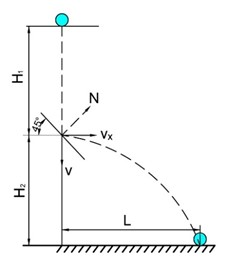Fig. 2. Principles of collision recovery coefficient measurement

The time for the sugarcane leaf to reach the collision plate in free fall from height H1 is t1. The height of the collision point between the sugarcane leaf and the 45° steel plate from the horizontal contact surface is H2. After the collision with the collision plate, the motion of the sugarcane leaf is parabolic, and the horizontal distance of the sugarcane leaf was L. The horizontal velocity after the collision is vx. The time for the sugarcane leaf to reach the horizontal contact surface is t2. The time to reach the horizontal contact surface is t2. At the collision recovery coefficient between the sugarcane leaves and the 45° steel collision plate could be calculated by Eq. 1,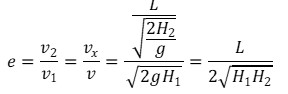(1)

where v, vx, v1, v2 can be obtained by applying Eqs. 2 to 6.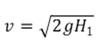(2)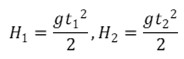(3)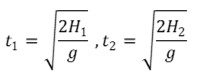(4)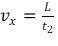(5)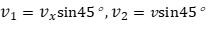(6)

Unlike traditional spherical and quasi-spherical materials, sugarcane leaves are flaky-shaped and leaf-shaped materials, along with smaller individual objects, including spherical particles such as seeds. This means that a certain drop height is required during their initial free fall in order to consider the effect of air resistance and the rebound effect of collision with the 45° steel collision plate. According to the ratio of the number of shredded sugarcane leaves to finely shredded sugarcane leaves, 13 shredded sugarcane leaves and 2 finely shredded sugarcane leaves were randomly selected in each group. The test process was recorded by a high-speed camera model SH6-202 (Shenyang Xingmai Technology Co., Ltd., Shenyang, China). After several repeated tests, it was determined that H1 and H2 were equal, that is, 223 mm.

When measuring the collision recovery coefficient between sugarcane leaves, the measured sugarcane leaves were prone to angular deflection when colliding with the sugarcane leaves on the 45° steel collision plate, so glue could be used to paste the sugarcane leaves on the 45° steel collision plate to reduce the measurement error.

After five trials, it was found that because of the large difference in the number of samples between the two tests and the random nature of the tests, the measurement differences between the two had less impact on the final measured results. Also, it was found that the results of crushed and finely crushed sugarcane leaves had similar parts at a certain point during the collision recovery coefficient measurement tests. Also considering the rigor of the measurement test, this work delineated the range for their measurement results. (Measurement of sliding friction coefficient and rolling friction coefficient was also the same). The experimentally measured collision recovery coefficients between sugarcane leaves and steel plates ranged from 0.1 to 0.26, and the collision recovery coefficients between sugarcane leaves were obtained from 0.05 to 0.2.

Friction Coefficient Measurement

Static friction coefficient measurement

The static friction coefficient between the sugarcane leaves and the steel plate, and between the sugarcane leaves and the sugarcane leaves could be measured by using a self-restraint steel inclinometer. The measurement principle is shown in Fig. 3(a). The procedure was to slowly raise the steel inclinometer until the sugarcane leaf was observed to have a sliding tendency on the steel plate determination plane, stop the action, record the angle indicated by the angle gauge on the inclinometer at this point, and record it as φ1. The static friction coefficient of sugarcane leaf and a steel plate was calculated using the formula. To determine the static friction coefficient between the sugarcane leaves, it was only necessary to use glue to paste the sugarcane leaves on the bevel of the steel plate, place the sugarcane leaves on the sugarcane leaves, and repeat the above action. After 5 sets of tests, the range of static friction coefficients between sugarcane leaves and steel plates was obtained from 0.2 to 0.7, and the range of static friction coefficients between sugarcane leaves was 0.25 to 0.5. The static friction factor can be calculated by Eq. 7,

μ1=tanφ1      (7)

where μ1 is the coefficient of static friction, and φ1 is the angle indicated by the angle gauge when the sugarcane leaf is about to slide on the steel inclinometer (°).

Rolling friction coefficient measurement

The dynamic friction factor of sugarcane leaves is difficult to measure and almost impossible to measure in its original state, so it was necessary to change its external form by making the original sheet-like appearance into a hollow cylindrical shape and placing it on a steel inclinometer. The inclinometer was turned slowly counterclockwise, and the angle measuring device above the inclinometer indicating the angle when it started to roll was recorded as φ2, as shown in Fig. 3(b). At this time the sugarcane leaves, when subjected to gravity, undergo a rolling process down the test ramp. By the law of conservation of energy, Eq. 8 can be obtained,

W=Ep Ek     (8)

where W is the energy lost in rolling of the sugarcane leaf (J), Ep is the initial gravitational potential energy of the sugarcane leaf (J), and Ek is the kinetic energy of the sugarcane leaf at the moment of termination (J).

Because the mass of a single sugarcane leaf was relatively small, the kinetic energy of its rolling down to the bottom of the test plane was difficult to obtain, the rolling friction coefficient obtained by approximate energy conservation within the initial rolling moment was used in this test to predict the true value (Ma et al. 2020). The force analysis on the inclinometer was as shown in the figure. When a sugarcane leaf with gravity G rolled at a tiny angle, the distance rolled across the plane of determination of the steel plate was S, and the positive pressure of the sugarcane leaves on the measurement plane was N=Gcosφ2. The rolling friction (F=μ2N) did work as W=FS, and the gravitational potential energy was Ep=GSsinφ2. Because the velocity of the hollow cylinder of the sugarcane leaf increases from zero within the tiny angle of the beginning of rolling, the velocity change was extremely small, and it was assumed that the kinetic energy of the sugarcane leaf was 0 at this time. From the above analysis, the rolling friction coefficient could be calculated by Eq. 9,

μ2=tanφ2     (9)

where μ2 is the coefficient of static friction, and φ2 is the angle indicated by the angle gauge when the sugarcane leaf is about to slide on the steel inclinometer (°).

To determine the rolling friction coefficient between the sugarcane leaves, the same as above could be done by pasting the sugarcane leaves to the steel plate. After 5 sets of tests, the range of static friction coefficient between sugarcane leaves and steel plate was determined to be in the range 0.05 to 0.3, and the range of static friction coefficient between sugarcane leaves was obtained as 0.05 to 0.3.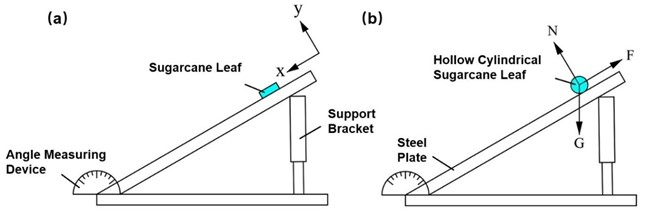Fig. 3. Friction coefficient of sugarcane leaves: (a) Sliding friction coefficient; (b) Rolling friction coefficient

Calibration of Contact Parameters of Sugarcane Leaves

Two experimental methods for measuring the stacking angle were used to optimize the results of the Plackett-Burman test, the steepest slope test and the Box-Behnken optimization test. The contact parameters between the sugarcane leaves and between the sugarcane leaves and the steel plate were used as factors and the relative error between the measured and simulated stacking angles as indicators to obtain the simulated contact parameters of the sugarcane leaves.

Actual stacking test

There are various complex states of motion during the formation of the accumulation angle of the bulk material, which are related to the physical properties of the contact material and the material itself, so it can be applied to the contact parameter calibration test of the discrete element model (Hamzah and Omar 2018; Torres-Serra et al. 2021). In this work, the stacking angle was measured by the cylinder lifting method and the injection funnel method based on existing studies. The universal testing machine and cylinder lifting experiment are shown in Fig. 4. The device consists of WDW-10 microcomputer-controlled electronic universal testing machine (Jinan Zhongbiao Instrument Co., Ltd., Jinan, China), a steel hollow cylinder, and a steel plate. The height of the steel hollow cylinder was 180 mm, and the inner diameter was 98 mm. The bottom of the cylinder initially rested on the surface of a 400 mm × 400 mm steel plate. The cylinder was filled with sugarcane leaves. A WDW-10 microcomputer-controlled electronic universal testing machine was used to lift the cylinder at a speed of 0.05 m/s. Sugarcane leaves were dropped on the steel plate out of the bottom of the cylinder, and the angle was measured by taking pictures after the sugarcane leaves were stabilized.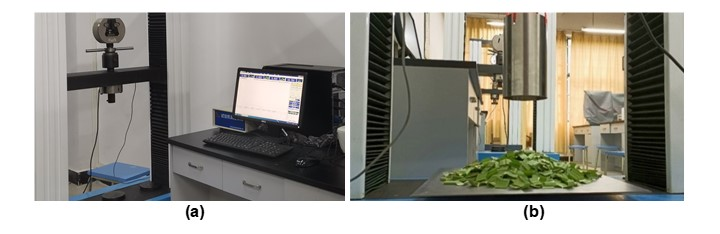Fig. 4. (a) Universal testing machine; (b) Cylinder lifting test to measure the stacking angle

The funnel test apparatus is shown in Fig. 5. The apparatus consists of a steel funnel with an outlet diameter of 55 mm and a steel plate with dimensions of 400 mm× 400 mm, where the distance between the outlet of the steel funnel and the steel plate was 200 mm. The sugarcane leaves used in the measurements were the same as those used in the cylinder lift test. The sugarcane leaves were slowly poured into the steel funnel and slightly stirred with a steel stirring bar to prevent the material from blocking the outlet, and the angle was measured by taking pictures after the sugarcane leaves were steadily discharged.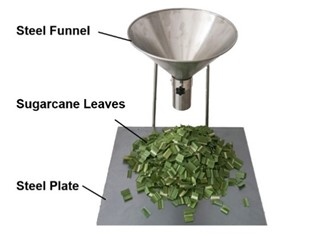Fig. 5. Injecting funnel method to measure the stacking angle

To avoid the errors caused by manual measurement, the collected images of the stacking angle α and β of the sugarcane leaf pile were denoised, grayscale processed, and binarized in turn using MatlabR2016a software (MathWorks Ltd., v.2016a, Natick, MA, USA) to obtain the boundary profile curve of the sugarcane leaf pile. Half of the curve edge contours were taken and imported into Origin 2017 software (Microcal, v.2017, Northampton, MA, USA), which was converted into coordinate data and linearly fitted using the software’s own image digitizing tool, and the obtained slope k was the angle value, as shown in Fig. 6. The above process was repeated five times for each of the two stacking angles to obtain the average values (Table 2). The determined stacking angles α and β of the sugarcane leaf pile were 26.3° and 31.6°, respectively.

Table 2. Measured Values of Sugarcane Leaf Stacking Angles α and β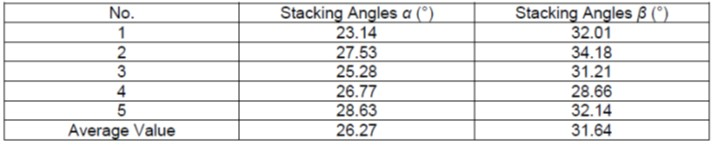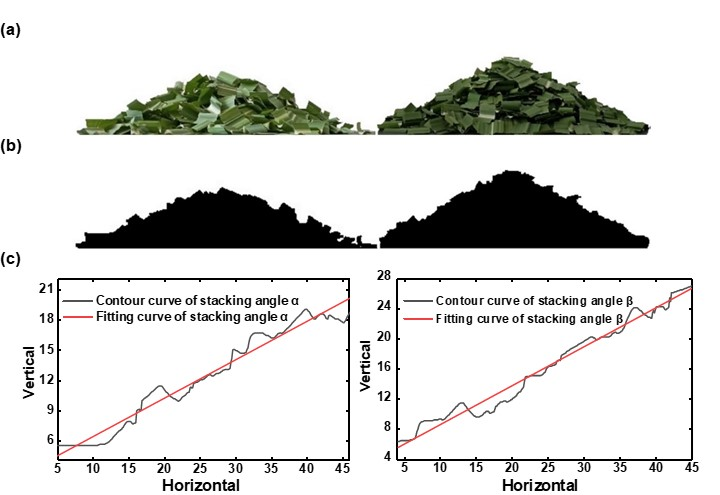Fig. 6. Image processing of sugarcane leaf pile-up angle: (a) Original image; (b) Binarized images; (c) Image processing

Simulation stacking test

The stacking angle simulation models were set up based on the actual parameters of the measured stacking angles. The two geometric models were established in a 1:1 ratio according to the measurement test requirements of two stacking angles α and β of sugarcane leaves. In this work, based on the physical test measurements and a review of the relevant literature (Hamzah and Omar 2018), the range of values of the necessary parameters for the discrete element simulation was known, as shown in Table 3. The sugarcane leaf particles and finely sugarcane leaf particles in the particle factory were separate, and the type of particle factory was set to dynamic factory. The production speed of shredded sugarcane leaves and finely shredded sugarcane leaves simulation model particles were set to 0.078 kg/s and 0.012 kg/s, and the production time was 2 s. Taking into account the feasibility of the test and the high efficiency of the software operation, the total time of both stacking angle simulations was set to 7 s, the time step was set to 25%, the calculation time step was 0.01 s, and the grid size was 3 x Rmin. The Hertz-Mindlin (no slip) contact model, which is commonly used in stacking angle simulation tests, was chosen for the simulation model. The two types of stacking angle simulation tests, cylinder lifting method and injection funnel method, are shown in Fig. 7.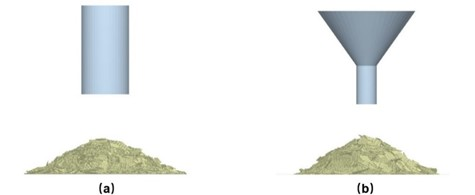Fig. 7. Simulated accumulation angle of sugarcane leaf: (a) cylinder lifting test; (b) injection funnel test

The image acquisition of the stacking angle was carried out at the end of the test, and the image was also processed using MatlabR2016a software (MathWorks Ltd., v.2016a, Massachusetts, USA) to obtain the simulated stacking angle θ and stacking angle γ. The stacking angle error Y1 and stacking angle error Y2 were calculated as Eq. 10.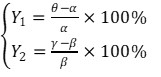(10)

RESULTS AND DISCUSSION

Plackett-Burman Test

Not all of the above measured parameters will necessarily have a significant effect on the relative error of the sugarcane leaf accumulation angle. To improve the accuracy of the final calibration parameters, it is necessary to screen out the parameters with significant effects (Torres-Serra et al. 2021). The Plackett-Burman test is able to select the high and low limit levels of each factor for a single-factor test under the conditions of simultaneous effects of multiple factors, so as to obtain the most significant effects parameter. In this work, calibration tests were conducted for the six physical parameters of sugarcane leaves measured above and the Poisson’s ratio of sugarcane leaves and the range of values of shear modulus of sugarcane leaves with reference to the relevant literature (Wen et al. 2020; Jiang et al. 2022; Lei et al. 2022; Tian et al. 2023). The range of each test parameter is shown in Table 2. The Plackett-Burman experimental design was applied to Design-Expert13 software (Stat-Ease, Minneapolis, MN, USA), and each group of experiments was repeated three times, averaged, and the corresponding relative errors were calculated. A total of 12 trials were conducted and the scheme and results are shown in Table 4.

Table 3. List of Plackett-Burman Test Parameters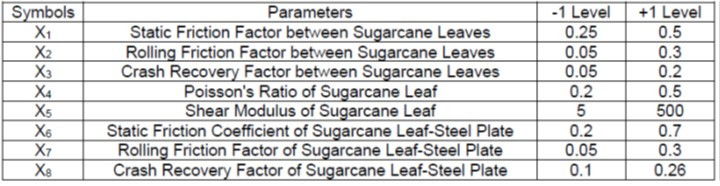Table 4. Protocol Design and Results of Plackett-Burman Test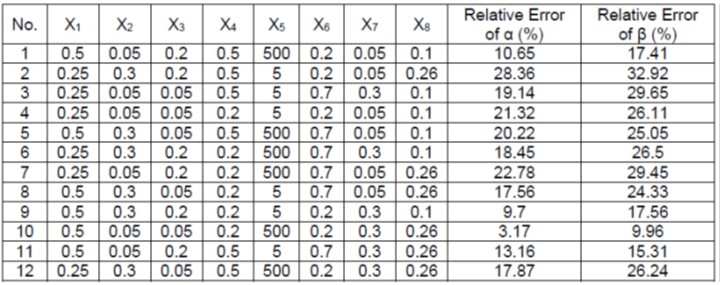Table 5. Significance Analysis of Plackett-Burman Test Parameters for Relative Error of α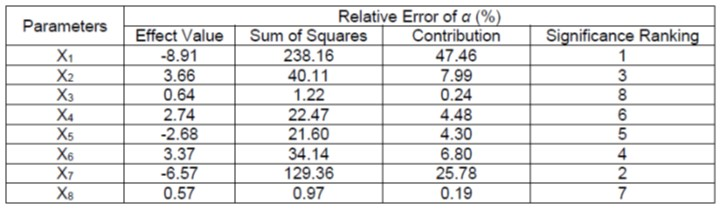As shown in Tables 5 and 6, the mass of a single sugarcane leaf was relatively small, and its kinetic energy of rolling down to the bottom of the test plane was difficult to obtain. The static friction factor between sugarcane leaves X1, the rolling friction factor between sugarcane leaves X2 and the rolling friction factor between sugarcane leaf-steel plate X7 were found to have significant effects on the two stacking angle errors, while the remaining parameters had less effects. Therefore, the parameters of X1, X2 and X7 were optimized in the steepest climb test as well as the orthogonal test.

Table 6. Significance Analysis of Plackett-Burman Test Parameters for Relative Error of β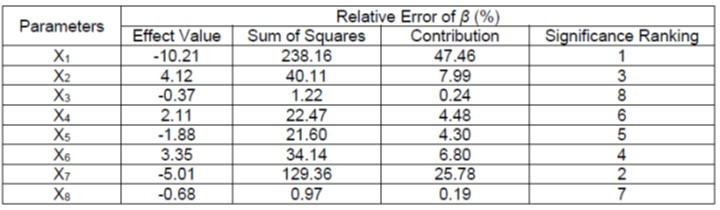Steepest Climb Test

If the optimal values of the parameters are within the selected range of high and low levels, the response surface analysis method (RSM) can be applied to build a regression model to solve for the optimal values (Zhang et al. 2022). The steepest climb test can determine the interval where the optimal values of factors are located relatively quickly (Tian et al. 2023), and its design scheme and results are shown in Table 7. The level intervals corresponding to the Plackett-Burman test screening significance influenced X1, X2, and X7 were divided into six equal parts, and the non-significant parameters were taken as intermediate values. The simulation tests for the two stacking angles were carried out, wherein each group of tests was repeated three times. The average values were taken, and the corresponding relative errors were calculated. The upper and lower limits of the RSM tests were determined with the relative error values of the two stacking angles of sugarcane leaves as the target.

Table 7. Steepest Climb Test Design and Results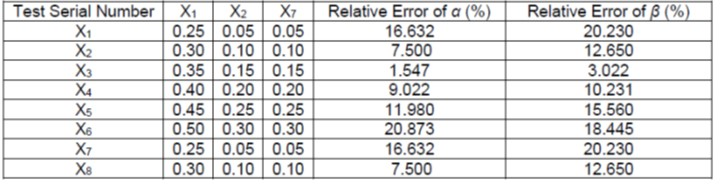Box-Behnken Test and Analysis of Significant Contact Parameters

Analysis of Table 7 indicates that the errors of the two stacking angles show a trend of decreasing and then increasing, with the smallest relative error in the No. 3 stacking angle. Based on the steepest climbing test, it was determined that each parameter in test No. 3 was used as the center point of the later test, and No. 2 and No. 4 were used as the low and high positions. Response surface methodology (RSM) was used to verify the significance relationship of the model and the optimal combination of parameters was derived by optimization.

Box-Behnken Test

The response surface experimental design was carried out using Design-Expert 13 software. A total of 17 sets of simulations were conducted, and the experimental design scheme and results are shown in Table 8. The regression model ANOVAs are shown in Tables 9 and 10.

Table 8. Box-Behnken Experimental Design Scheme and Results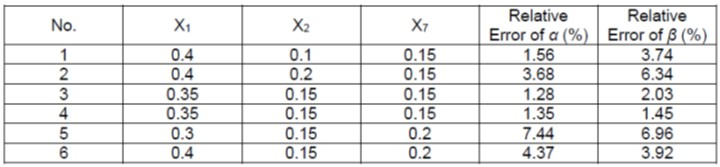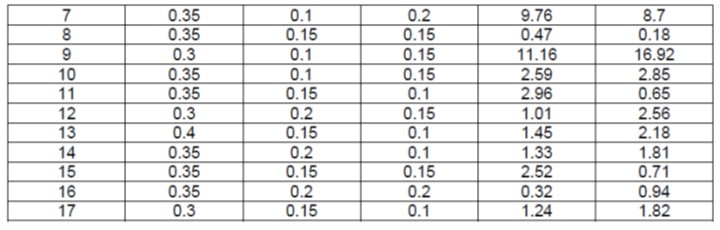Table 9. Box-Behnken Test Regression Model Analysis of Variance for Relative Error of α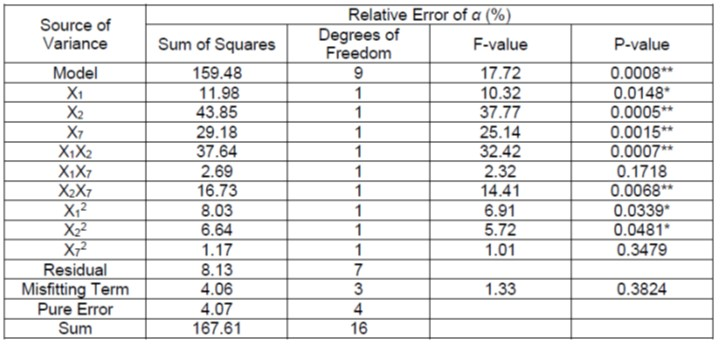As shown in Table 9, the regression model was highly significant (P<0.01), which could reliably reflect the relationship between the independent and dependent variables, and the misfit term of the model was not significant (P>0.05), indicating that the regression equation was a good fit and there was no influence of irrelevant factors (Yuan et al. 2018; Geng et al. 2021). In addition, the effect of independent variables X1, X12, and X22 on the relative error of stacking angle α was significant (P<0.05), the effect of independent variables X2, X7 and interaction factors X1X2 and X2X7 on the relative error of stacking angle α was highly significant (P<0.01), and all other terms were insignificant.

Multiple regressions were fitted to the Box-Behnken test results, and the relative errors of the sugarcane leaf piling angle α were obtained with the second-order regression equations of the three significance parameters as shown in Eq. 11.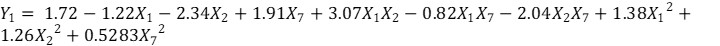(11)

As shown in Table 10, the regression model for β was also highly significant (P<0.01), and the misfit term of the model was not significant (P>0.05), indicating that the fit of the regression equation was good and there was no influence of irrelevant factors. In addition, the effect of the interaction factor X2X7 on the relative error of the stacking angle β was significant (P<0.05), the effect of the remaining factors on the relative error of the stacking angle β was highly significant (P<0.01), and all other terms were not significant.

Table 10. Box-Behnken Test Regression Model Analysis of Variance for Relative Error of β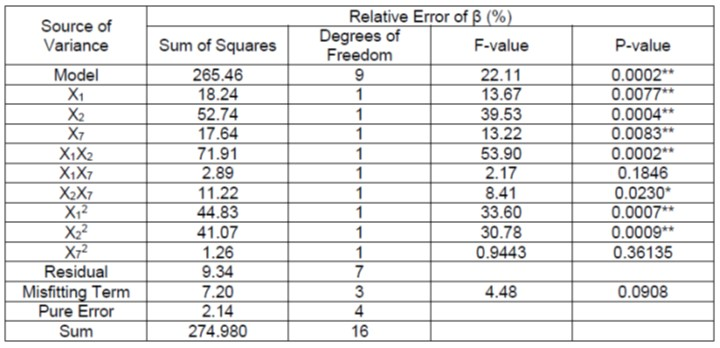The relative error of the sugarcane leaf piling angle β was obtained with the second-order regression equation of the three significance parameters as shown in Eq. 12.